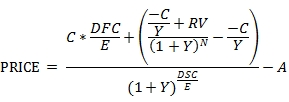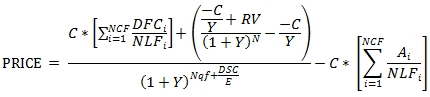# SQL Server pricing function for odd-first coupon bonds

OFC

Updated: 31 May 2014

Use OFC to calculate the price or yield of a bond with an odd first period and a par value of 100. The OFC formula for a bond with an odd short first coupon is:Where
A = C * accrued days / E
C = 100 * coupon rate / frequency
DFC = the number of days from the issue date to the first coupon date
DSC = number of days from settlement to coupon
E = the number of days in the quasi-coupon period
N = the number of coupons between the first coupon date and the maturity date
RV = redemption value
Y = yield / frequency
The OFC formula for a bond with an odd long first coupon is:Where
Ai = number of accrued days for the ith quasi-coupon period
C = 100 * coupon rate / frequency
DFCi = number of days from the issue date to the first quasi-coupon date (i=1) or the number of days in the quasi-coupon period (i>1).
DSC = number of days from settlement date to the next quasi-coupon date or first coupon date.
E = number of days in the quasi-coupon period in which settlement occurs
N = the number of coupons between the first coupon date and the maturity date
NCF = number of quasi-coupon periods that fit in the odd period
NLFi = normal length in days of the full ith quasi-coupon period within the odd period.
Nqf = the number of whole quasi-coupon periods between the settlement date and the first coupon.
RV = redemption value
Y = yield / frequency

The OFC function allows you to pass value for DFC1, DFC2, A1, A2, NLF1, NLF2, NCF, N, NqF, DSC, E, and RV directly into the equation and automatically calculates Y and C. OFC does not support bonds with more than 2 quasi-coupon periods.
Syntax
SELECT [wctFinancial].[wct].[OFC](
<@Rate, float,>
,<@Yield, float,>
,<@Price, float,>
,<@RV, float,>
,<@Freq, int,>
,<@E, float,>
,<@DSC, float,>
,<@N, int,>
,<@ShortFirst, bit,>
,<@A1, float,>
,<@DFC1, float,>
,<@NLF1, float,>
,<@A2, float,>
,<@DFC2, float,>
,<@NLF2, float,>
,<@Nqf, int,>)
Arguments
@Rate
the security’s annual coupon rate. @Rate is an expression of type float or of a type that can be implicitly converted to float.
@Yield
the security’s annual yield. @Yield is an expression of type float or of a type that can be implicitly converted to float.
@Price
the price of the bond. @Price is an expression of type float or of a type that can be implicitly converted to float.
@RV
the security’s redemption value per 100 face value. @RV is an expression of type float or of a type that can be implicitly converted to float.
@Freq
the number of coupon payments per year. For annual payments, @Freq = 1; for semi-annual, @Freq = 2; for quarterly, @Freq = 4; for bimonthly @Freq = 6; for monthly @Freq = 12. @Freq is an expression of type float or of a type that can be implicitly converted to float.
@E
the number of days in the settlement period. @E is an expression of type float or of a type that can be implicitly converted to float.
@DSC
the days from settlement to next coupon date (when @N > 1) or the days from settlement to redemption (when @N = 1). @DSC is an expression of type float or of a type that can be implicitly converted to float.
@N
the number of coupons from the first coupon date to the maturity date. @N is an expression of type int or of a type that can be implicitly converted to int.
@ShortFirst
a bit value which identifies the bond as having a short first coupon period ('True') or a long first coupon period ('False'). @ShortFirst is an expression of type bit or of a type that can be implicitly converted to bit.
@A1
the number of accrued days in the first quasi-coupon period. @A1 is an expression of type float or of a type that can be implicitly converted to float.
@DFC1
the number of days from the issue date to the quasi-coupon date (when @ShortFirst = 'False') or the number of days from the issue date to the first coupon date (when @ShortFirst = 'True'). @DFC1 is an expression of type float or of a type that can be implicitly converted to float.
@NLF1
the normal length of the first quasi-coupon period. @NLF1 is an expression of type float or of a type that can be implicitly converted to float.
@A2
the number of accrued days in the second quasi-coupon period. If @ShortFirst = 'True' then @A2 should be NULL .@A2 is an expression of type float or of a type that can be implicitly converted to float.
@DFC2
the number of days from in the second quasi-coupon period. If @ShortFirst = 'True' then @DFC2 should be NULL. @DFC2 is an expression of type float or of a type that can be implicitly converted to float.
@NLF2
the normal length of the second quasi-coupon period. If @ShortFirst = 'True' then @NLF2 should be NULL. @NLF2 is an expression of type float or of a type that can be implicitly converted to float.
@Nqf
the number of whole coupon periods between the settlement date and the first coupon date. If @ShortFirst = 'True' then @Nqf should be 0. @Nqf is an expression of type int or of a type that can be implicitly converted to int.
Return Type
float
Remarks
·         If @Rate is NULL then @Rate = 0.
·         If @RV is NULL then @RV = 100.
·         If @Freq is NULL then @Freq = 2.
·         If @E is NULL then @E = 180.
·         If @DSC is NULL then @DSC = 0.
·         If @N is NULL then @N = 0.
·         If @ShortFirst is NULL then @ShortFirst = 'True'.
·         If @A1 is NULL then @A1 = 0.
·         If @DFC1 is NULL then @DFC1 = 0.
·         If @NLF1 = 0 then @NLF1 = @E.
·         If @A2 is NULL then @A2 = 0.
·         If @DFC2 is NULL then @DFC2 = 0.
·         If @NLF2 is NULL then @NLF2 = 0.
·         If @Nqf is NULL then @Nqf = 0.
·         If @Yield is NULL and @Price is NULL then NULL is returned.
·         If @E = 0 then NULL is returned.
·         If @Freq = 0 then NULL is returned.
·         C = 100 * @Rate/@Freq
·         Y = @Yield/@Freq
·         If @Yield is NOT NULL then price is calculated from the inputs otherwise yield is calculated from the inputs.
Examples
This bond has an odd short first coupon (meaning that the first coupon period is shorter than a normal coupon period) and settles on the issue date.
SELECT
wct.OFC(
0.025,       --@Rate
0.0276,      --@Yield
NULL,        --@Price
100,         --@RV
2,           --@Freq
182,         --@E
45,          --@DSC
40,          --@N
'True',      --@ShortFirst
0,           --@A1
45,          --@DFC1
NULL,        --@NLF1
NULL,        --@A2
NULL,        --@DFC2
NULL,        --@NLF2
NULL         --@Nqf
) PRICE
This produces the following result.
PRICE
----------------------
96.0075631077824
This bond has odd long first coupon (meaning that the first coupon period is longer than a normal coupon period) and settles on the issue date.
SELECT
wct.OFC(
0.025,       --@Rate
0.0276,      --@Yield
NULL,        --@Price
100,         --@RV
2,           --@Freq
182,         --@E
45,          --@DSC
39,          --@N
'False',     --@ShortFirst
0,           --@A1
45,          --@DFC1
182,         --@NLF1
0,           --@A2
183,         --@DFC2
183,         --@NLF2
1            --@Nqf
) PRICE
This produces the following result.
PRICE
----------------------
96.0033702877755
Here we calculate the yield of a bond with an odd short first coupon.
SELECT
wct.OFC(
0.0257,      --@Rate
NULL,        --@Yield
98.116208,   --@Price
100,         --@RV
2,           --@Freq
181,         --@E
15,          --@DSC
41,          --@N
'True',      --@ShortFirst
14,          --@A1
29,          --@DFC1
NULL,        --@NLF1
NULL,        --@A2
NULL,        --@DFC2
NULL,        --@NLF2
NULL         --@Nqf
) YIELD
This produces the following result.
YIELD
----------------------
0.0268999998598751
Here's an example of the price calculation with a negative yield.
SELECT
wct.OFC(
0.0157,      --@Rate
-0.00235,    --@Yield
NULL,        --@Price
100,         --@RV
2,           --@Freq
180,         --@E
15,          --@DSC
21,          --@N
'True',      --@ShortFirst
14,          --@A1
29,          --@DFC1
NULL,        --@NLF1
NULL,        --@A2
NULL,        --@DFC2
NULL,        --@NLF2
NULL         --@Nqf
) PRICE

This produces the following result.
PRICE
----------------------
119.276791530979

### SupportCopyright 2008-2021 Westclintech LLC         Privacy Policy        Terms of Service